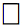Powerful Physics Software - Maplesoft

# Powerful Software for Physics Computations.By physicists, for physicists

So you can focus on your ideas, not the computations.

## Maple Physics Package

Maplesoft provides the essential math environment for scientific research. By combining the world’s most advanced symbolic computation engine with powerful numeric algorithms, advanced visualization tools, and intuitive interfaces, Maplesoft products are helping physicists around the world deepen our understanding of how the universe works.

### Maple has the most comprehensive support for physics of any mathematical software package.

Because of the frequent use of anti-commutative and non-commutative variables, functions, vectors, tensors and matrices; specialized rules and operators; and extremely complex notation, algebraic computations in physics are a serious challenge for most mathematical software. While some specialized systems handle a small fraction of this domain, Maple is the only system that provides the ability to handle a wide range of physics computations as well as pencil-and-paper style input and textbook-quality display of results. In addition, the Physics package is an integral part of the entire Maple system, so using Maple for physics also gives you access to Maple’s full mathematical power, programming language, visualization routines, and document creation tools.

The section below lists Maple’s capabilities in algebraic computations in physics. In every case, problems in that area can be expressed in Maple using the same notation as you would use when writing the problem on paper, and the results are displayed in the same way as they would be shown in a textbook.

### Maple’s capabilities in algebraic computations in physics

#### Vector Analysis

• Projected vectors
• Non-projected abstract vectors
• Cartesian, cylindrical, and spherical algebraic unit vectors
• Projected vectorial differential operators (e.g. Nabla)
• Abstract vectorial differential operators
• Scalar and cross product operators for vectors that handle projected and abstract vectors
• Differentiation taking into account relationships between geometrical coordinates
• Supporting vectorial routines for changing basis, representing components of abstract vectors, etc.

#### Tensor Analysis (n-dimensional Geometry, Special and General Relativity)

• User defined tensors (under addition, multiplication, differentiation, simplification, and transformation of coordinates)
• Distinction between covariant and contravariant indices
• User defined systems of coordinates, possibly many at the same time
• Coordinate transformation of tensorial expressions taking into account covariant and contravariant indices
• Computation of components of arbitrary tensorial expressions
• Determination of free and repeated indices, simplification and differentiation using Einstein's sum rule
• Complete set of general relativity tensors
• Searchable database of solutions to Einstein's equations

#### Product Operators

• Standard multiplication operator (*) handling commutative, anticommutative and noncommutative variables in equal footing
• Automatic normal form for products involving commutative, anticommutative, and noncommutative variables
• Scalar and cross product operators for 3-D vectors and vectorial functions or vectorial differential operators
• Scalar product operator for Bras and Kets and related quantum state (Dirac notation) vector calculus

#### Differential Equations Support

• Computation of Poincare sections for dynamical systems
• Reducing, triangularizing, and/or solving DE systems, possibly  involving anticommutative variables and functions
• Symmetry analysis for ODEs and PDEs, possibly including anticommutative variables and functions

#### Quantum state vector calculus (Dirac’s notation)

• Bras and Kets
• Projectors
• Annihilation/Creation operators
• Orthonormal discrete and continuous bases
• Hermitian and non-Hermitian operators, possibly tensorial
• Pauli and Dirac matrices including their commutator algebras and the computation of traces of products
• Commutators and anticommutators
• Customizable commutator and anticommutator algebra rules
• Simplification taking everything into account (fixed and customizable algebra rules, Einstein's sum rule, etc.)

#### Field Theories (Classical and Quantum)

• Ability to represent Lagrangians and Hamiltonians involving anticommutative and noncommutative variables, functions and operators
• Ability to compute field equations using standard and functional differentiation
• Ability to compute the analytic structure of the Feynman Diagrams of a model

#### Differentiation Operators

• Differentiation with respect to anticommutative variables, scalar and tensor functions
• Functional differentiation with respect to commutative and anticommutative scalar and tensor functions
• The d’Alembertian, the galileanand covarianttensorial differentiation operators

#### Differential Geometry Support

• Calculus on manifolds (vector fields, differential forms and transformations)
• Tensor analysis in advanced general relativity
• Calculus on jet spaces
• Lie algebras
• Lie and transformation groups
• Computations can be performed in user-specified frames
• Computations can be performed with abstract differential forms (coordinate-free)

User Case Study